Wei, X., Xu, S., Sun, L., Tian, C., and Du, C. (2021). "Propagation velocity model and two-dimensional defect imaging of stress wave in larch (Larix gmelinii) wood," BioResources 16(4), 6799-6813.

#### Abstract

The propagation law of stress wave in larch (Larix gmelinii) wood was studied in this work. External factors affecting the propagation velocity of stress wave in wood cross-section were studied using the orthogonal experiment method. The most influential factors were shown by the experimental results, and the parameters of the propagation velocity model of stress wave in larch wood were optimized. Based on the optimized propagation velocity model, combined with the traditional defect determination method, a twelve-directional stack imaging (TDSI) steps system was developed for larch wood internal defect detection. The analysis results showed that of the three external factors of temperature, moisture content, and illumination duration, temperature had the greatest influence on the propagation velocity model of stress wave in larch wood cross-section. Using TDSI to image the defective larch wood not only can locate the defective area, but also it can achieve a high imaging precision of 95.52%, and the imaging precision is unrelated to the location of the defect, which has a good quantitative defect detection effect.

Propagation Velocity Model and Two-dimensional Defect Imaging of Stress Wave in Larch (Larix gmelinii) Wood

Xiwen Wei,a,b Shuzheng Xu,a Liping Sun,a,* Cong Tian,b and Chunxiao Du c

The propagation law of stress wave in larch (Larix gmelinii) wood was studied in this work. External factors affecting the propagation velocity of stress wave in wood cross-section were studied using the orthogonal experiment method. The most influential factors were shown by the experimental results, and the parameters of the propagation velocity model of stress wave in larch wood were optimized. Based on the optimized propagation velocity model, combined with the traditional defect determination method, a twelve-directional stack imaging (TDSI) steps system was developed for larch wood internal defect detection. The analysis results showed that of the three external factors of temperature, moisture content, and illumination duration, temperature had the greatest influence on the propagation velocity model of stress wave in larch wood cross-section. Using TDSI to image the defective larch wood not only can locate the defective area, but also it can achieve a high imaging precision of 95.52%, and the imaging precision is unrelated to the location of the defect, which has a good quantitative defect detection effect.

Keywords: Stress wave; Orthogonal experiment method; Temperature; Twelve-directional stack imaging; Defect imaging

Contact information: a: College of Mechanical and Electrical Engineering, Northeast Forestry University, Harbin, 150040, China; b: College of Electrical and Information Engineering, Heilongjiang University of Technology, Jixi, 158100, China; c: Harbin Veterinary Research Institute, Chinese Academy of Agricultural Sciences, Harbin, 150040, China; *Corresponding author: zdhslp@163.com

INTRODUCTION

The stress wave technique has been widely used in nondestructive testing of wood because of its low cost, portability, and the fact that it is harmless to human beings (Wang et al. 2008; Yue et al. 2017; Zhang and Khoshelham 2020). Yang and Wang (2007) used the Arbotom to detect the internal decay of wood, and they compared and analyzed the actual estimated value with the estimated value of the internal decay area of wood. The test results indicated that two-dimensional images of the interior decay from wood could be obtained with the non-destructive test technology of stress wave, but the measuring accuracy is low. Li et al. (2014) examined stress wave velocity patterns in the cross-sections of black cherry trees, developed analytical models of stress wave velocity in sound healthy trees, and then tested the effectiveness of the models as a tool for tree decay diagnosis. With the rapid development of computer technology, many researchers have begun to use some algorithms to realize the images of wood internal defects. Du et al. (2018) proposed an image reconstruction method based on segmented propagation rays of stress waves to detect the size and shape of defects inside wood. The results showed that the proposed algorithm not only can better reflect internal wood defects qualitatively and quantitatively, but also it has a high quality of reconstructed images. Qiu et al. (2019) developed a tomographic technique based on the use of both stress wave and electromagnetic wave for evaluating the defects in tree trunk, the result indicated that the internal air hole and the air gap located within 5 to 20 mm below the tree surface can be effectively detected and quantified.

However, the propagation of stress waves is also affected by various factors in wood, and it has become one of the main topics for researchers to explore (Liu et al. 2014; Chen et al. 2019). Xu and Wang (2011) studied the effects of moisture content and temperature on the propagation velocity of stress waves in Korean pine wood. The work showed that moisture content is an important factor affecting wave propagation, and a regression model was established between the propagation velocity of stress wave and moisture content. Liu and Gao (2014) examined the effects of moisture content and density on the stress wave velocity in wood. For the same wood species, a good positive linear correlation between the stress wave velocity and the basic density was found under any moisture content. However, there was no similar correlation among different wood species, which might be due to the differences in the compositions. Yamasaki et al. (2017) investigated the effect of moisture content on the stress wave propagation velocity and obtained the relationship between the moisture content and the rate of change of velocity of full-size timber. This relationship was used to estimate the Young’s modulus of the timber in the air-drying state from the velocity in high-moisture condition.

Recently, most of the research on wood nondestructive testing of stress wave mainly has focused on the establishment of propagation velocity model, the influencing factors of propagation velocity, and the imaging method. However, the study of the influence degree of various factors on the stress wave propagation velocity affecting the change of the stress wave propagation velocity model has not been reported. This paper explores the propagation laws of stress wave in healthy and defective wood cross-sections, and it establishes an experimental model of stress wave propagation velocity and the chord angle of healthy larch wood samples under different influence factors of moisture content, temperature, and illumination duration by orthogonal experiment. According to the orthogonal experimental results, the most influential factors of stress wave propagation in samples are obtained, and the procedure optimizes the experimental model. Based on the optimized propagation velocity model, a new imaging algorithm was designed, and it was compared with the traditional algorithm to achieve quantitative analysis of wood internal defects. This paper provides a new theory for the most influential factors of the propagation velocity of larch wood and two-dimensional defect imaging.

Theoretical Analysis of Stress Wave Propagation Velocity in Wood

Theoretical model of stress wave propagation velocity in healthy wood

For simplicity, it is assumed that the cross-section of an ideal wood piece is a regular circle, as shown in Fig. 1(a), where S represents the position of the stress wave emitting end sensor, R and F represent the position of the stress wave receiving end sensor, and a represents the chord angle between the SR and the SF, which is negative clockwise and positive counterclockwise. Generally, the radial and tangential tensile strength of healthy wood is lower, and the radial propagation velocity is the fastest, and it decreases with the increase of the absolute value of a (Wei et al. 2020).

According to Dikrallah et al. (2006), the acoustic anisotropy of wet wood was analyzed using the guided wave experiment, and the propagation rule of stress wave in the cross-section of wood was studied. The mathematical relationship between stress wave propagation velocity V and the chord angle a is obtained as follows,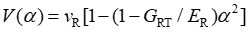(1)

where V(a) is the propagation velocity of stress wave in cross-section (m/s), vR is radial velocity (a = 0), and ER and GRT represent tangential modulus of elasticity (MPa) and shear modulus (MPa), respectively.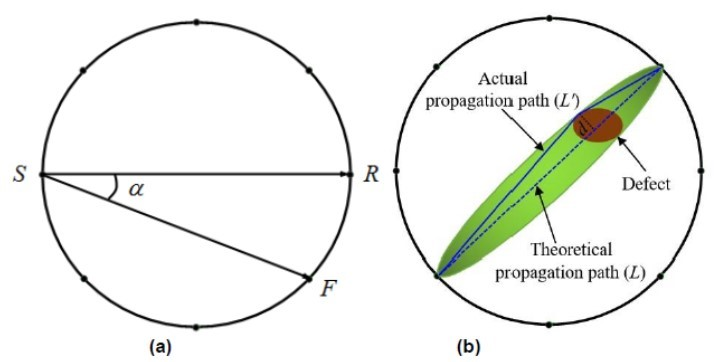Fig. 1. Schematic diagram of stress wave propagation in (a) healthy wood and (b) defect wood

VR, GRT, and ER are determined by the nature of the wood itself, the relationship between the stress wave propagation velocity, and the chord angle is similar to a downward parabola. Equation 1 is taken as the basic model of the stress wave propagation velocity in healthy wood cross-section.

Modified model of stress wave propagation velocity in defective wood

Stress waves travel more slowly in defective wood due to the presence of defect area. Such area causes a propagating wave to deviate from a straight path, increasing the transmission time between transmitters and receivers, as shown in Fig. 1(b). Assuming that the actual propagation path length is L‘ , the theoretical propagation path length is L, the longest distance from the defect edge to the theoretical path is d, the theoretical propagation path is divided into two parts (l1 and l2). According to the Pythagorean Theorem, the following mathematical relation can be obtained, as represented by Eq. 2: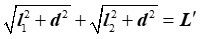(2)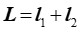(3)

According to Eqs. 2 and 3, Eq. 4 can be obtained: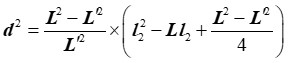(4)

Equation 4 can be transformed into Eq. 5: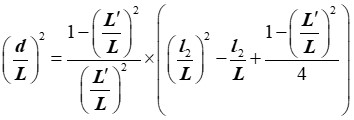(5)

The propagation path correction angle of the defect edge is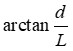. In order to ensure that the correction angle does not exceed the actual defect range, the minimum value of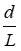is taken as follows: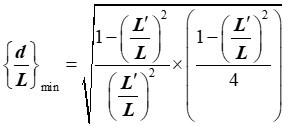(6)

The ratio of the theoretical propagation path length to the actual propagation path length is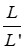, and the ratio of theoretical propagation velocity to actual propagation velocity of stress wave is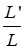. Therefore, the correction angle of the actual propagation direction can be calculated using the theoretical propagation velocity and the actual propagation velocity, and the closer the defect area is to the edge of the sample, the higher the correction accuracy.

EXPERIMENTAL

Materials and Equipment

Table 1 lists the basic information of five healthy larch (Larix gmelinii) wood samples (Northeast Forestry University, Harbin, China) L1 to L5, among which samples L1 to L4 were applied to orthogonal design experiment, and the sample L5 was used for the model optimization experiment.

Table 1. Basic Situation of Wood Samples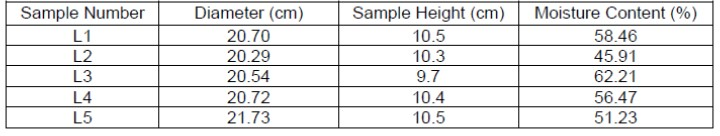In the experiment, the moisture content of wood was measured via the drying measure method with a 202-0 desktop drying box (Beijing Yongguangming Medical Instrument Co., Ltd., Beijing, China), the propagation velocity was measured using the stress wave tester (Arbotom, Frank Rinn, Heidelberg, Germany), the high and low temperature test chamber (MINCHANG instrument and equipment Co., Ltd., Xiamen, China) was used to adjust the temperature of the sample, and the digital thermometer GM1361 (BENETECH, Shenzhen Jumaoyuan Technology Co., Ltd., Shenzhen, China) was used to measure the indoor, freezer, and the samples’ temperature.

Methods

Orthogonal experimental design

In the research of nondestructive testing technology of stress wave wood, wood temperature, moisture content, and illumination duration are the main external factors that affect the propagation velocity of stress wave, and they also have a great influence on the accuracy of the collected data of stress wave propagation velocity. To obtain the optimal model of stress wave propagation velocity in wood under the influence of three factors, this paper used the orthogonal experiment method to collect stress wave propagation velocity data in wood cross-sections under different conditions of temperature, moisture content, and illumination duration, and performed the regression analysis with the theoretical model as objective function. At the same time, the goodness of fit was analyzed by the range analysis method. The influence degree of each factor on the fitting result was judged, and the propagation velocity model was optimized. In this paper, an orthogonal experimental design with two levels and three factors was adopted (Nha et al. 2020). The experimental factors and levels are shown in Table 2. Two levels of temperature were set as 10 °C and 20 °C, respectively. Two levels of moisture content were dry state and green state, respectively. Two levels of illumination duration were 0 h and 72 h, respectively.

Table 2. Orthogonal Experiment Factors and Levels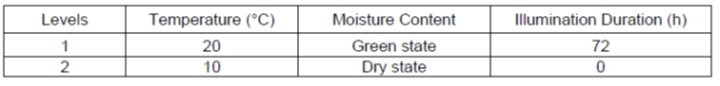Four groups of experiments were randomly generated by orthogonal design software (SPSS Statistics 26.0, IBM Corp., Armonk, NY, USA), and the resulting experimental design is shown in Table 3. The sample in experiment 1 was L1, which was green state under 20 °C and 72 h of light. The sample L2 in experiment 2 was an absolutely dry state under the condition of 20 °C and 0 h of light. The sample L3 in experiment 3 was green state at 10 °C for 0 h. The sample L4 in experiment 4 was dry state with 10 °C and 72 h of illumination.

Table 3. Orthogonal Experiment Plan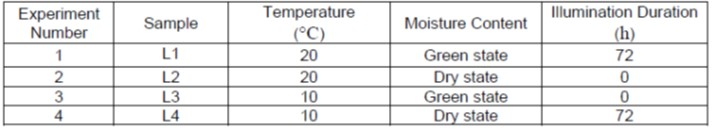Determination of moisture content and temperature

In order to reduce the effects of variations in sample moisture content, the sample was packaged during transportation. It was transported back to the laboratory at an ambient temperature of 23 °C and air relative humidity of 54% for 48 h. In the above orthogonal experimental design, the moisture content level of samples L1 and L3 were set to the green state, so a representative moisture content test piece with a thickness of 1 cm was sawed at the end of the sample. The rest were sealed with plastic bags. The moisture content level of samples L2 and L4 were set to the absolute dry state, so the samples were directly used as the test piece for measuring the moisture content, so that the moisture content can be measured and dried to the absolute dry state. Using the drying method, the weight was regarded as initial mass (m), and the test piece was then dried in a constant temperature oven at 103 ± 2 °C for about 24 h. The weight was taken out and recorded, and then returned to the oven for further drying. It was weighed every 4 h until the weight of the last two weights was constant. The final weight was recorded as the absolute dry weight (m0) of the test piece. The formula for calculating the moisture content of L1 to L4 was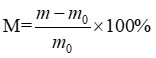, and the absolute dry state samples L2 and L4 were sealed with plastic bags.

Then, the samples L1 and L2 were placed in the temperature test chamber with set temperature of 20 °C for 12 h, and the samples L3 and L4 were placed in the temperature test chamber with set temperature of 10 °C for 12 h. The digital thermometer GM1361 was inserted the samples to test the actual temperature, and the difference between the actual samples temperature and the set temperature was within ± 0.3 °C. To avoid the change of the internal temperature and moisture content of the samples during the measurement, the samples were taken out of the test chamber and measured quickly, and the measurement time was controlled within 60 s each time.

Sample L5 was used for the optimization experiment of stress wave propagation velocity model. First, the stress wave test was carried out on sample L5 at normal temperature (23 °C), and then it was put into the temperature test chamber with the temperature set at 2 °C, 4 °C, 6 °C, 8 °C, 10 °C, 12 °C, 14 °C, 16 °C, 18 °C, and 20 °C, respectively. In order to prevent the sample from absorbing water or evaporation of internal water, it was sealed with a plastic bag. At each temperature stage, the sample was placed for 12 hours, and the temperature difference was within 0.3 °C. The specimen was taken out to be able to measure the propagation velocity of stress wave within 60 s. After the test, the specimen was put it into the test chamber quickly and the next test was carried out.

Stress wave velocity measurement

In the four groups of experimental environments, the propagation velocity of cross-section stress wave was measured for each experimental sample. The cross-section of larch sample was approximately regarded as a circle. The circumference of the circle was calculated through the diameter, and then the circle was divided into twelve equal parts. Twelve sensors were arranged on twelve equal points of the cross-section, as shown in Fig 2. During the experiment, each sensor was used as the transmitting end in turn to collect data, and finally, the stress wave propagation velocity at the same chordwise angle was summed and averaged as the stress wave propagation velocity at the chordwise angle. The theoretical propagation velocity model was taken as the objective function for regression analysis, and the determination coefficient of fitting results was output as each experimental result for analysis.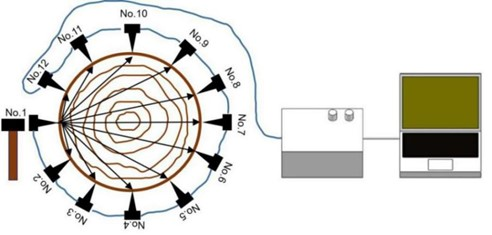Fig. 2. Stress wave nondestructive testing of wood cross-section

Image reconstruction algorithm

Related research (Jiao et al. 2018; Liu and Li 2018) shows that the cross-section of wood, when the measured wave propagation velocity on a certain propagation path is more than 10% different from the theoretical value, can be considered the path that passes through the defective area. Considering this issue, a modified image reconstruction method is presented in this paper. Using the velocity calculated by the established stress wave propagation velocity model as the theoretical value, a twelve-directional stack imaging method (TDSI) was designed. According to the stress wave measurement method, the speed of wood with defects was collected, and the healthy area and the defective area were marked with different colors to realize two-dimensional imaging. The specific steps were as follows:

Step 1: A defective sample was selected to determine the approximate detection range. Twelve sensors were uniformly arranged on the cross-section of the sample, which were marked as No.1 to No.12 in turn. Each one was used as transmitting ends, and other sensors were used as receiving ends to collect data in 12 directions.

Step 2: The cross-section of the sample was approximately regarded as a circle, and the measured values of each round were processed separately. The actual measured value of each transmission route was compared with the health reference value of the model, and if the difference was more than 10%, it was regarded as abnormal and marked.

Step 3: After marking all the propagation paths, each propagation path is judged. If two adjacent propagation paths were abnormal, all the enclosed areas were defined as defect areas. If two adjacent propagation paths were both healthy paths, then the area enclosed by them was marked as a healthy area. If one of the two adjacent propagation paths was abnormal and the other was healthy, it was concluded that the defect boundary was between the two propagation paths. The abnormal propagation path was corrected to the defect boundary by using the correction Eq. 6, and the area surrounded by the abnormal propagation path before correction and the abnormal propagation path after correction was all marked as a defect area.

Step 4—Repeat steps 2 and 3 and test all the sensors in turn to overlay 12 imaging sub-images. The rule is to divide all the images into 3000 points. If all points in the same position of the 12 sub-images are marked as defect points, then the point is marked as the defect point in the final imaging image, otherwise the point is marked as a healthy point. Lastly, a final two-dimensional imaging map aft superposition is obtained.

Other analytical methods

Origin software (OriginLab, v.9.0, Northampton, MA, USA) was used to draw the relationship between stress wave propagation velocity and chord angle. The twelve-directional stack imaging method (TDSI) and the cross-detection imaging method (CDIM) use the open source web application, Jupiter Notebook, and programs are written in Python language.

RESULTS AND DISCUSSION

Results Based on Experiment Data of Wood

Take the average value of stress wave propagation velocity at different chordwise angles of cross-section in the above four groups of experiments, as shown in Table 4. The propagation velocity of stress wave increased first and then decreased with the increase of chord angle in each group of experiments, which is consistent with the theoretical Eq. 1. However, the propagation velocity of stress wave at the same chord angle was quite different under different moisture content, different temperature, and different illumination duration, which indicates that external factors greatly influence the propagation velocity of stress wave.

Table 4. Propagation Velocity of Stress Wave in Cross-section of Larix Under Different Experiment Conditions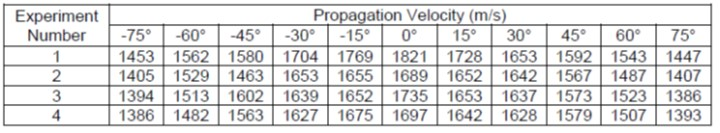The relationship between stress wave propagation velocity and chord angle in each group of experiments is visually shown in Fig. 3, and the regression analysis is performed with theoretical model as objective function (Table 5). The determination coefficient of fitting function is quite different when external factors and conditions are different. Under the influence of external factors, such as temperature, moisture content, and illumination duration, the propagation velocity of stress wave in larch wood cross-section is affected to some extent, and the regression analysis results of stress wave propagation velocity collected under various conditions are also different. The regression analysis results of the four groups of experiments, the quadratic coefficient of the fitting function was approximately -0.05, and the constant term was approximately 1700. However, the goodness of fit of regression analysis differed among the four groups of experiments. Experiment 4 had the best goodness of fitting, with a determination coefficient of 0.99, and experiment 2 had a determination coefficient of only 0.87, which indicates that the propagation velocity of stress wave in wood will have a certain deviation from the theoretical model under the influence of external conditions.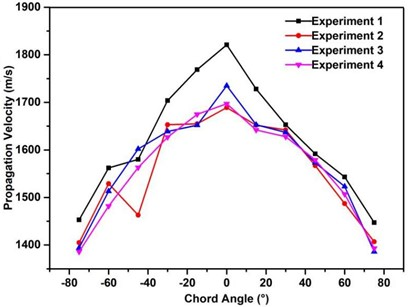Fig. 3. Diagram of stress wave propagation velocity in cross section of larix under different experiment conditions

Table 5. Regression Model of Stress Wave Propagation Velocity and Chord Angle Under Different Experiment Conditions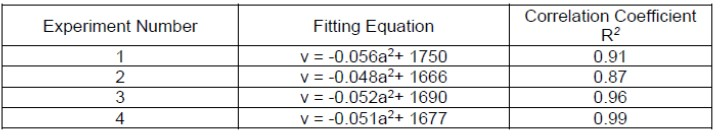Analysis of influencing factors and model optimization

In order to further understand the relationship between horizontal factors and investigation indicators, this experiment calculates the extreme difference Rj, that is, the difference between the maximum value and the minimum value of the results indicators of factors in the experimental horizontal interval. According to the size of Rj, each factor that affects the experimental results indicators can be ranked. A large value indicates that the factor has a great effect on the experimental results, whereas a small value indicates that the corresponding factor has a smaller effect on the experimental results, and its calculation formula is (Yao et al. 2021):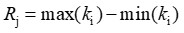(7)

In the formula, Rj is the extreme difference value, and max(ki) and min(ki) are the maximum and minimum values, respectively, of the result indexes of the factors in the experimental horizontal interval.

In the orthogonal experiment results, the extreme difference of temperature factor was 0.085, moisture content was 0.005, and illumination duration was 0.035. It is apparent that among the three external factors, temperature, moisture content, and illumination duration, temperature had the greatest influence on the propagation velocity model of stress wave in wood cross-section. In order to collect stress wave propagation velocity at suitable temperature, the stress wave propagation velocity of wood sample L5 was measured at 2 °C, 4 °C, 6 °C, 8 °C, 10 °C, 12 °C, 14 °C, 16 °C, 18 °C, and 20 °C, and the results are shown in Table 6.

Table 6. Propagation Velocity of Stress Wave in Different Temperatures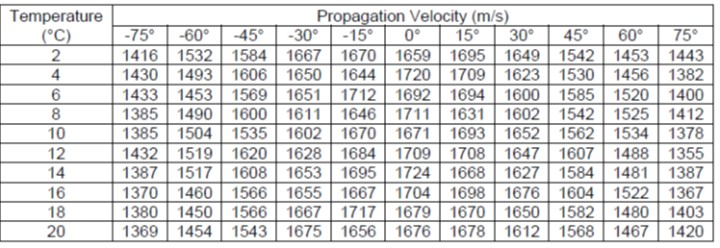Table 6 shows that the temperature was changed and the change amplitude of stress wave propagation velocity at different chordwise angles was inconsistent. This further indicates that the change of temperature alters the data of stress wave propagation velocity collected during actual detection, thus affecting the accuracy of theoretical propagation model.

The stress wave propagation velocity was analyzed with the theoretical model as the objective function, and the results are shown in Table 7. According to Table 7, when the temperature ranged from 2 °C to 20 °C, the coefficient of quadratic term of fitting function in regression analysis ranged from -0.059 to -0.047, and the constant term ranged from 1664 to 1706. The determination coefficients R2 were all above 0.9, and the significance of P was less than 0.001. The results were consistent with the theoretical model.

Table 7. Regression Analysis of Stress Wave Propagation Velocity in Different Temperatures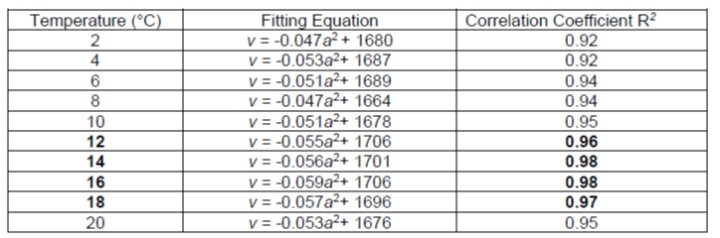In Table 7, with the increase of temperature, the determination coefficients of the fitting equations first increased and then decreased. When the temperature was 10 °C, the determination coefficient was 0.95. From 12 to 18 °C, the determination coefficients were greater than 0.95, and the determination coefficient decreased to 0.95 of 20 °C. Therefore, the fitting equations were selected to obtain the optimization model in the range of 12 to 18 °C. In this temperature range, the quadratic coefficient of the fitting function ranged from -0.059 to -0.055, and the constant term ranged from 1696 to 1706, with a small error range. The formula of the relationship between the propagation velocity v of the final stress wave on the cross-section of healthy larch and the chordwise angle was obtained by averaging the coefficients of the functions with the fitting function determination coefficient above 0.95 in the regression analysis results.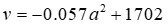(8)

This formula, as the theoretical optimal model of stress wave propagation velocity of larch, was used to calculate the health reference value.

Defect image analysis

According to TDSI steps, the wood samples with artificial defects were reconstructed, and the samples are approximated as regular circles as imaging. At the same time, to compare with other stress wave tomography algorithms, this paper repeats the cross-detection imaging method (CDIM) proposed by Weng et al. (2016). The experimental results of contrast imaging for N1 to N3 defect samples are shown in Figs. 4 to 6.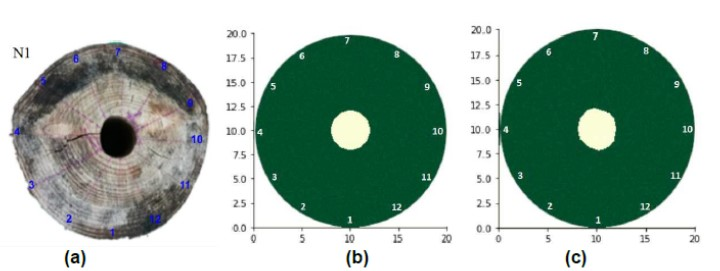Fig. 4. (a) Sample N1, (b) tomographic image of CDIM, and (c) tomographic image of TDSI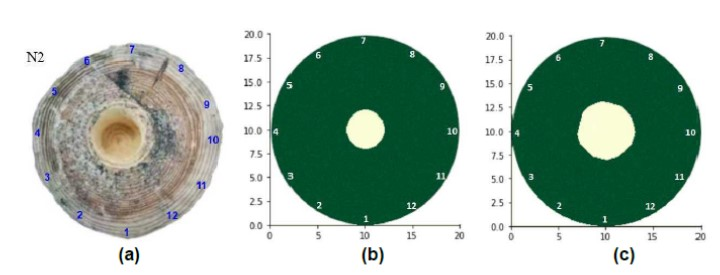Fig. 5. (a) Sample N1, (b) tomographic image of CDIM, and (c) tomographic image of TDSI

The defect position of the sample N1 is in the center of the log, and its shape is oval, as shown in Fig. 4(a). The two imaging algorithms were more accurate in defect location. In the absence of propagation path angle correction formula, the defect area restored by the final imaging result was approximately a symmetric circle (Fig 4. (b)), and the defect shape restored in the final imaging image was similar to the actual defect shape due to the addition of propagation path direction angle correction link in the TDSI.

The defect position of the sample N2 was also in the center of log, and its shape was round, as shown in Fig. 5(a). The two algorithms were more accurate in the initial location of defects. The defect in the CDIM was obviously smaller than that in the TDSI and the actual image, and the shape of defect was highly similar to that of N1. Although the shape of defect in the imaging image of TDSI was an irregular circle, it was similar to the actual shape of defect. The reasons are as follows. Because the angle between two adjacent paths of stress wave in 11 propagation paths of sample cross-section is 15° in each round of CDIM, the algorithm colors the propagation paths passing through the defect area. However, when the defect area covers the propagation path with an angle of 15° to the radial direction but does not cover the propagation path with an angle of 30° to the radial direction, then the final imaging results are the same regardless of the size and shape of the defect area change. Therefore, although the defect diameter of N2 was larger than that of N1, the result of two-dimensional imaging by the CDIM was the same. The best way to solve this problem is to increase the number of sensors as much as possible to increase the coverage density of the propagation path and reduce the errors caused by the imaging algorithm.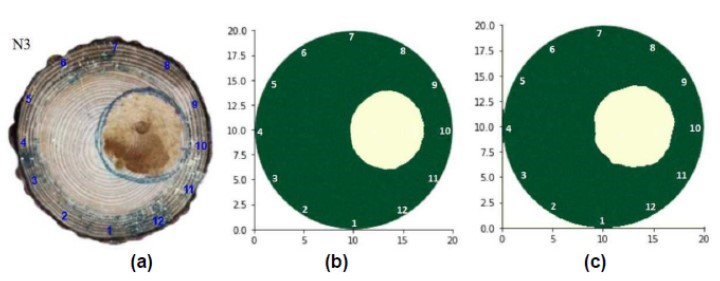Fig. 6. (a) Sample N1, (b) tomographic image of CDIM, and (c) tomographic image of TDSI

The defect shape of N3 sample was approximately circular, but the edge of defect was irregular. Compared with N1 and N2, the defect position was in the right half of the sample, as shown in Fig. 6(a). The two algorithms were both accurate in the initial location of defects. The defect in the CDIM was a circle, and the irregular defect edge could not be restored. The restoration of the right half was roughly accurate, while the left half was not accurate. The TDSI was able to restore its defect shape accurately. The reason was analyzed. Because the defect location was on the right side of the sample, in the CDIM, the propagation path covered by the right half defect area was denser than that of the left half defect area, and the probability of the propagation path participating in the final defect area crossing the defect edge was greatly improved. The imaging error of the right half defect is smaller than that of the left half. In the TDSI, every round of imaging reconstructs the defect area, and after twelve rounds, the defect will be more obvious and accurate, so the imaging effect has nothing to do with the position of the defect.

The defect area of the actual measurement S and the defect area calculated by the two algorithms S1 and S2 are shown in Table 7. The parameters delta S1 and delta S2 represent the imaging precision of the CDIM and the TDSI, respectively, and they can be used to verify the correctness of the test results. The formula is as follows: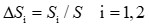(9)

Based on the data of Table 8, the results indicate that both methods underestimated the size of the real defects. The imaging precision range of CDIM was between 68.33% and 98.37%, and the imaging precision range of TDSI was between 90.27% and 95.52%. The average imaging precision of CDIM was 85.2%, while the average imaging precision of TDSI was 92.16%. According to the results of the experiment, in the CDIM, each propagation path with a radius of 15° goes through the defect edge of sample N1, and the shape of the defect edge happens to be circular, so the imaging accuracy is high. The imaging process was similar to that of sample N1, the defect area of sample N2 was slightly larger than that of sample N1, and the accuracy of defect detection immediately decreased. However, in the TDSI, the imaging precision of the defect area was all greater than 90%, when the defect position deviates from the center of the cross-section, the imaging precision of TDSI was also larger than that of CDIM. It has been shown that TDSI has a higher imaging precision, this imaging algorithm can accurately detect the location and size of internal defects in logs, and it also can provide a theoretical and experimental basis for further realization of 3D imaging.

Table 8. The Results of Defective Area Detection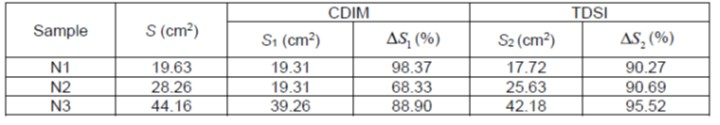CONCLUSIONS

1. Propagation laws of stress wave in healthy wood cross-section were theoretically analyzed. An experimental model of stress wave propagation velocity and the chord angle of larch wood samples under different moisture content, temperature, and illumination duration was established by orthogonal experiment.
2. Among the external factors affecting the propagation of stress wave in larch wood, temperature had the greatest influence on the propagation velocity of stress wave, and the extreme difference of orthogonal experimental results reached 0.085, which was greater than the extreme difference of moisture content (0.005) and illumination duration (0.035). On the condition that other factors are determined, the propagation velocity of stress wave at different temperatures was measured. The regression analysis results show that the actual propagation velocity of stress wave at 12 to 18 °C was in best accord with the theoretical model, and the optimal propagation velocity model of stress wave in larch wood cross-section was obtained: v = -0.057a2 + 1702.
3. The propagation law of stress wave in defective wood was analyzed, and the correction model of stress wave propagation angle in the process of defect imaging was established. Based on the optimized propagation velocity model, combined with the traditional defect determination method, the paper has presented a twelve-directional stack imagine (TDSI) system for larch wood internal defect detection.
4. The TDSI achieved an accurate effect on the quantitative detection of defects, and the two-dimensional imaging precision of defects reached over 90.27%, which is 6.96% higher than the average accuracy of the cross-detection imaging method (CDIM). When the defects were at the edge of the cross-section, the imaging accuracy reached 95.52%. The TDSI has a high degree of defect shape restoration and can accurately detect the position and shape of void defects on wood cross section.

ACKNOWLEDGMENTS

The authors are grateful for the support of the Natural Science Foundation of Heilongjiang Province of China (Grant No. LH2020C091), and the 2020 Special Foundation Project of Fundamental Scientific Research Professional Expenses for Undergraduate Universities in Heilongjiang Province (Grant Nos. 2020-KYYWF-0514, 2020-KYYWF-0516, and 2020-KYYWF-0511)

REFERENCES CITED

Chen, W., Zeng, H., Gao, Y., Zhao, T., Zhou, Y., and Hao, Q. (2019). “Analysis on the influence factors of stress wave propagation velocity of Casuarina equisetifolia and two kinds of acacia standing trees,” Journal of Hainan Normal University (Natural Science) 32(3), 281-287. (In Chinese)

Dikrallah, A., Hakam, A., Kabouchi, B., Brancheriau, L., Bailleres, H., Famiri, A., and Ziani, M. (2006). “Experimental analysis of acoustic anisotropy of green wood by using guided waves,” in: Proceedings of the Joint Meeting of the ESWM and COST Action E35, Florence, Italy, pp. 149-154.

Du, X. C., Feng, H. L., Hu, M. Y., Fang, Y. M., and Chen, S. Y. (2018). “Three-dimensional stress wave imaging of wood internal defects using TKriging method,” Computers and Electronics in Agriculture 148, 63-71. DOI: 10.1016/j.compag.2018.03.005

Jiao, Z., Li, G., and Wu, X. (2018). “Tomography imaging algorithm based on velocity error correction for stress wave nondestructive evaluation of wood,” Journal of Beijing Forestry University, 40(01), 108-119. (In Chinese) DOI: 10.13332/j.1000-1522.20170384

Li, G., Wang, X., Feng, H., Wiedenbeck, J., and Ross, R. J. (2014). “Analysis of wave velocity patterns in black cherry trees and its effect on internal decay detection,” Computers and Electronics in Agriculture 104, 32-39. DOI: 10.1016/j.compag.2014.03.008

Liu, L., and Li, G. (2018). “Acoustic tomography based on hybrid wave propagation model for tree decay detection,” Computers and Electronics in Agriculture 151, 276-285. DOI: 10.1016/j.compag.2018.06.020

Liu, H., Gao, J., Chen, Y., and Liu, Y. (2014). “Effects of moisture content and fiber proportion on stress wave velocity in cathay poplar (Populus cathayana) wood,” BioResources 9(2), 2214-2225. DOI: 10.15376/biores.9.2.2214-2225

Liu, H., and Gao, J. (2014). “Effects of moisture content and density on the stress wave velocity in wood,” Journal of Beijing Forestry University 36(6), 154-158. (In Chinese) DOI: 10.13332/j.cnki.jbfu.2014.06.002

Nha, V., Goos, P., and Schoen, E. D. (2020). “Integer programming approaches to find row-column arrangements of two-level orthogonal experimental designs,” IISE Transactions 52(7), 780-796. DOI: 10.1080/24725854.2019.1655608

Qiu, Q., Qin, R., Lam, J. H. M., Tang, A. M. C., Leung, M. W. K., and Lau, D. (2019). “An innovative tomographic technique integrated with acoustic-laser approach for detecting defects in tree trunk,” Computers and Electronics in Agriculture 156, 129-137. DOI: 10.1016/j.compag.2018.11.017

Wang, L., Xu, H., Yan, Z., Lv, J., Yang, X., Lv, J., Yang, X., and Zhou, C. (2008). “Effects of sensor quantity and planar distribution on testing results of log defects based on stress wave,” Scientia Silvae Sinicae 44(5), 115-121. (In Chinese)

Weng, X., Li, G., Feng, H., Du, X. C., and Chen, F. (2016). “Stress wave propagation velocity model in RL plane of standing trees,” Scientia Silvae Sinicae 52(7), 104-112. (In Chinese)

Wei, X., Sun, L., Zhou, H., Yang, Y., Wang, Y., and Gao, Y. (2020). “Propagation velocity model of stress waves in larch wood (Larix gmelinii) three-dimensional space with different moisture contents,” BioResources 15(3), 6680-6695. DOI: 10.15376/biores.15.3.6680-6695

Xu, H. D., and Wang, L. H. (2011). “Effects of moisture content and temperature on propagation velocity of stress waves in Korean pine wood,” Scientia Silvae Sinicae 47(9), 123-128. (In Chinese)

Yang, X., and Wang, L. (2007). “Gain and analysis of two-dimensional images of interior decay of logs with stress wave method,” Scientia Silvae Sinicae 43(11), 93-97. (In Chinese)

Yamasaki, M., Tsuzuki, C., Sasaki, Y., and Onishi, Y. (2017). “Influence of moisture content on estimating Young’s modulus of full-scale timber using stress wave velo-city,” Journal of Wood Science 63(3), 225-235. DOI: 10.1007/s10086-017-1624-5

Yao, L., Ma, R., and Wang, H. (2021). “Baidu index-based forecast of daily tourist arrivals through rescaled range analysis, support vector regression, and autoregressive integrated moving average,” Alexandria Engineering Journal 60(1), 365-372. DOI: 10.1016/j.aej.2020.08.037

Yue, X., Wang, L., Wang, X., Rong, B., Ge, X., Liu, Z., and Chen, Q. (2017). “Quantitative detection of internal decay degree for standing trees based on three NDT methods-electric resistance tomography, stress wave imaging and resistograph techniques,” Scientia Silvae Sinicae 53(3), 138-146. (In Chinese)

Zhang, J., and Khoshelham, K. (2020). “3D Reconstruction of internal wood decay using photogrammetry and sonic tomography,” Photogrammetric Record 35(171), 357-374. DOI: 10.1111/phor.12328

Article submitted: June 4, 2021; Peer review completed: August 10, 2021; Revised version received and accepted: August 15, 2021; Published: August 24, 2021.

DOI: 10.15376/biores.16.4.6799-6813